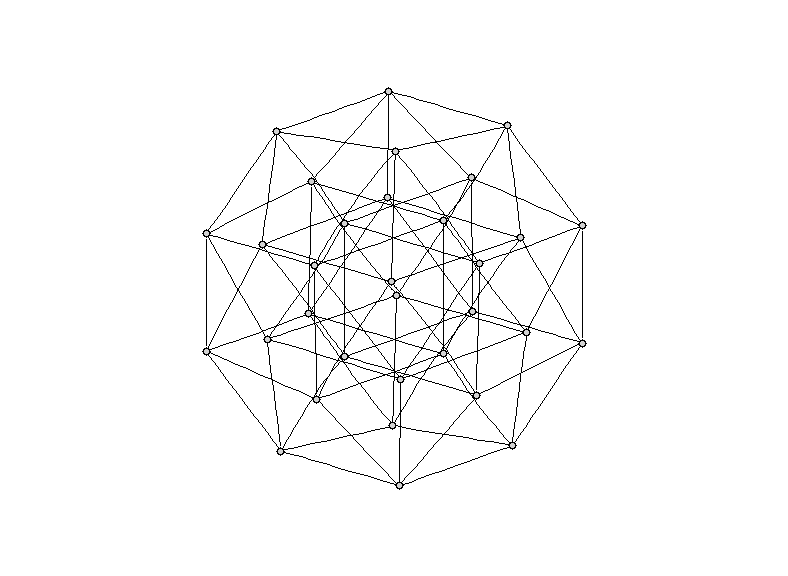# mathr / blog / #

## Drawing polytopes with Haskell and GraphVizSo I was toying with using Graphviz to render polytopes (higher-dimensional generalisations of polygons and polyhedra). I quickly got bored writing .dot files by hand, and switched to the Haskell programming language.

Here's some code that generates a .dot file containing the vertices and edges of a cube of arbitrary dimension:

> module Main where
>
> import System(getArgs)
>
> type Node = [Bool]
>
> edges node = filter (\(u,v) -> u /= v) $zipWith (\n b -> (n, zipWith (||) n b)) (repeat node) bits > where > bits = map bit [0..(n-1)] > bit b = map (== b) [0..(n-1)] > n = length node > > nodes 0 = [[]] > nodes d = concatMap (\n -> [False:n, True:n]) ns where ns = nodes (d-1) > > main = do > args <- getArgs > let d = read (head args) :: Int > e = concatMap edges (nodes d) > putStrLn ("strict graph Cube" ++ (show d) ++ "D {") > putStrLn " node [label=\"\",shape=\"circle\",width=0.1,height=0.1,fixedsize=true,style=\"filled\"];" > sequence (map printEdge e) > putStrLn "}" > where > printEdge (u,v) = putStrLn (" " ++ showNode u ++ " -- " ++ showNode v ++ ";") > showNode n = "p" ++ map showCoord n > showCoord True = '1' > showCoord False = '0' Above is the output for a cube of 5 dimensions, rendered with neato from Graphviz. I also wrote code for the cross polytope (in 3 dimensions it's an octahedron): > module Main where > > import System(getArgs) > > type Node = Int > > edges d nodes node = filter (\(u,v) -> u < v && u /= (v + d) mod (2 * d))$ zip (repeat node) nodes
>
> nodes d = [0..(2*d -1)]
>
> main = do
>   args <- getArgs
>     showNode n = "p" ++ show n